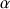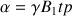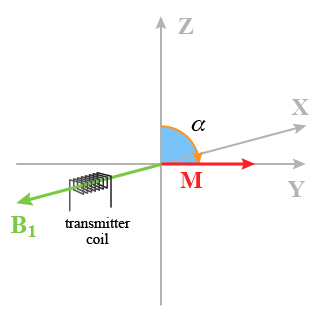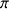# Two-dimensional NMR spectroscopy

## The alpha impulsion angle and the position of the M vector in a rotating coordinate system

Impulsion angledu vecteur M (Fig 5) and position of the M vector (Fig. 5) is given by the following relationwith B1 which represents the amplitude or the power of the impulsion and tp the width or duration of the impulsion. It is possible to make these two values vary together in such a way that particularly interesting rotation angles appear. One of these is the angle=90°.

In this case all the magnetization is orientated in the plane xy and the signal reaches its maximum of intensity.Fig. 5 : Location of the M vector after an impulsion time allowing an alpha angle of 90°

An other angle allows the inversion of the M vector by the application of an impulsionor= 180°

In this experiment, the vector M is orientated in –z. Practically, one uses the angle= 45° in order to get a good compromise between measurement time/quality of the response.

1. D.L. Rabenteck

Sensitivity enhancement by signal averaging in pulsed by Fourier transform NMR spectroscopy ; J. of Chem. Ed. Vol. 61, n°70, Oct 1984